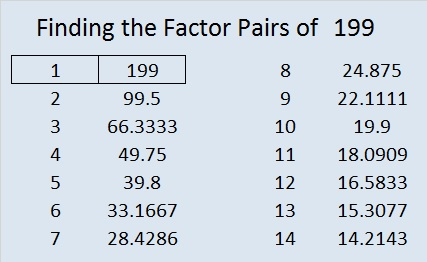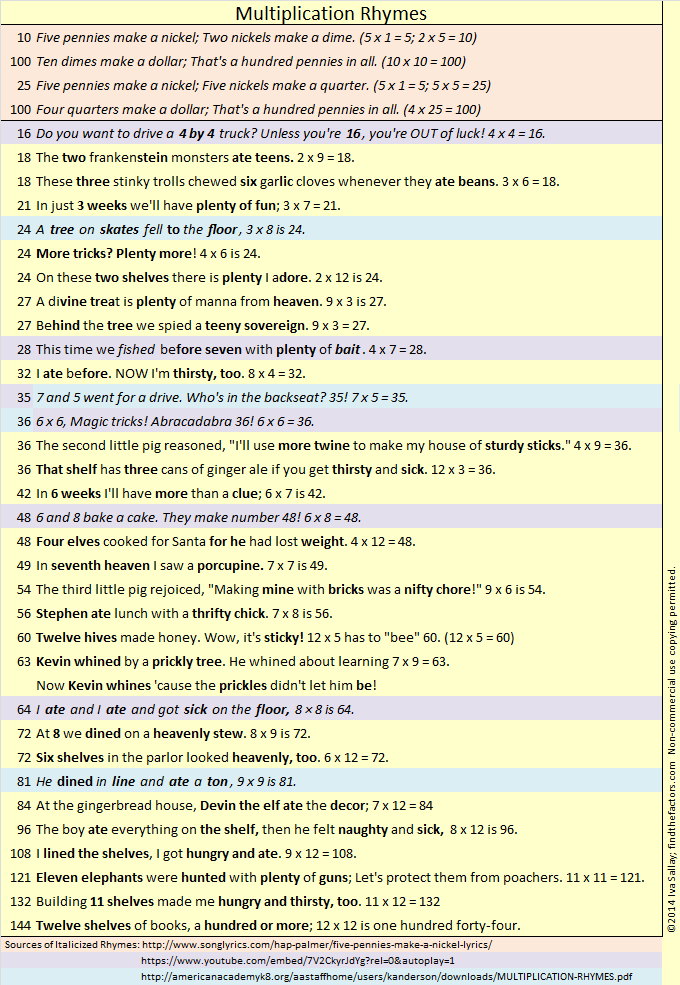# 199 My List of Multiplication Rhymes

• 199 is a prime number.
• Prime factorization: 199 is prime.
• The exponent of prime number 199 is 1. Adding 1 to that exponent we get (1 + 1) = 2. Therefore 199 has exactly 2 factors.
• Factors of 199: 1, 199
• Factor pairs: 199 = 1 x 199
• 199 has no square factors that allow its square root to be simplified. √199 ≈ 14.1067How do we know that 199 is a prime number? If 199 were not a prime number, then it would be divisible by at least one prime number less than or equal to √199 ≈ 14.1. Since 199 cannot be divided evenly by 2, 3, 5, 7, 11, or 13, we know that 199 is a prime number.

———————————

I recently wrote a post that asked “Are Multiplication Rhymes Able to Help Kids Learn the Multiplication Table?” Here is a list of rhymes that I’ve put together.Most of the rhymes have rhyming clues to help students remember both numbers being multiplied as well as their product. I wrote the rhymes that are highlighted in yellow. Those highlighted in peach, purple, or blue are from other sources, but I did alter the rhymes for 24, 28, and 81. A black and white pdf of the rhymes is also available here.

## One thought on “199 My List of Multiplication Rhymes”

1.Paula Beardell Krieg

What a great list of number rhymes! It’s awesome that you put these together in one place. Oh, and I like knowing that 199 is a prime. thank you.

This site uses Akismet to reduce spam. Learn how your comment data is processed.